## Introduction

Analysis of the isotopic composition of molecules is one of the key tools for studying element cycles on earth. For the light elements H, C, N and O with relatively small heavy-to-light isotope ratios at natural abundance, the standard analytical instruments have largely limited isotope analysis to single-substituted isotopocules (isotopically substituted molecules). Studies of multiply substituted isotopocules, referred to as clumped isotopes, were only occasionally carried out, often using isotopically enriched substrates or labeling experiments1,2,3,4,5. Recent analytical advancements using isotope ratio mass spectrometry6,7,8 or laser spectroscopy9 have enabled high precision measurements of clumped isotopes in several molecules such as CO2, CH4, O2 and N2O8,10,11,12,13,14,15 and the field is rapidly expanding.

Since multiply substituted isotopocules are thermodynamically more stable than single substituted ones, classical isotope theory predicts small but measurable positive clumped isotope anomalies for most molecules under natural conditions16,17,18,19. These clumped isotope signatures depend on temperature, which is the basis of the new field of clumped isotope thermometry20.

Yeung et al.14 and Wang et al.13 reported negative heavy isotope clumping in photosynthetic O2 formation and in biogenic CH4, respectively. Yeung et al.14 attributed the negative Δ values (see equation 3 for definition) in photosynthetic O2 to different isotopic composition of the two O atoms originating from different sites in the oxygen evolving complex of photosystem II. Triggered by this observation we investigated this further and show here that negative clumping anomalies are necessarily expected whenever two or more indistinguishable atoms of the same element but with different isotopic composition combine in a molecule. The atoms do not need to share a common bond but can be at distant places in a molecule.

Yeung21 recently presented an analysis of such apparent statistical clumped isotope effects in combination with other isotope effects. In our paper, we restrict the analysis to statistical clumped isotope effects and phrase the calculations exclusively in terms of isotope ratios in order to elucidate the underlying general nature of these apparent isotope signatures. The fundamental origin of the apparent statistical clumped isotope effect is thoroughly presented and visualized geometrically. We then provide a general mathematical formalism for apparent statistical clumped isotope signatures in any multiple isotope system. Finally we demonstrate quantitatively how a certain measured statistical anti-clumping signal can be used to determine the isotopic heterogeneity of indistinguishable atoms in a molecule.

## Origin of the statistical negative clumped isotope signatures

We describe and calculate the statistical clumped isotope effects in terms of heavy-to-light isotope ratios iR of individual atoms and molecules, where the index i indicates the mass of the atom or molecule. The same letter R is used for both atomic isotope ratios and molecular isotopocule ratios. In particular, for molecules with multiple heavy isotopes (clumped isotopes), the heavy-to light isotopocule ratio is defined asWhen two atoms with atomic heavy-to-light isotope ratios R1 and R2 (where Ri can be, e.g., 2H/1H, 13C/12C, 18O/16O, etc.) combine in a molecule in a purely random manner, i.e., without any isotope effect, the ratio of molecules that include the heavy isotopes of both of these atoms relative to molecules including only light isotopes is simply the product of the atomic isotope ratios of the two atoms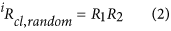Clumped isotope signatures Δi are then by convention calculated as the relative difference between a certain (measured) clumped isotopocule ratio and the random clumped isotope ratio, usually reported in per mill (‰).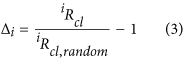The apparent negative statistical clumped isotope signatures that we describe in this paper are fundamentally related to this referencing convention, in particular the choice of the reference ratio iRcl,random that is required to calculate Δi in Eq. 3. When the isotope ratios R1 and R2 of the involved atoms are individually known, iRcl,random = R1 · R2 can be precisely calculated. This is always the case when heavy isotopes of different elements clump together (e.g. 13C and 18O in CO or in CO2). It also holds when molecules of the same element, for which the individual isotope ratios are known, clump together (e.g. 15Nα and 15Nβ in N2O, where α and β indicate the central and terminal position of the N atom in the linear NNO molecule, which can be determined independently22,23). This is graphically illustrated in Fig. 1, where R1 and R2 are plotted on the x- and y-axis and their product, iRcl,random, is shown as the blue area.

When a molecule contains indistinguishable atoms of the same element, it is impossible to determine the individual atomic isotope ratios of these atoms. Nevertheless, the atoms may originate from isotopically distinct populations with isotope ratios R1 and R2. To calculate the correct value of iRcl,random we would therefore need to know the individual isotope ratios R1 and R2. However, since these isotope ratios cannot be retrieved for indistinguishable atoms, it is common (and reasonable) to assign the bulk (≈average, see below) isotopic composition of the atoms. Through this choice, the real stochastic clumped isotope ratio R1R2 (blue area in Fig. 1) is substituted by the approximated value RavRav (red area in Fig. 1). Both areas have the same perimeter 2  (R1 + R2) =2  (Rav + Rav), but the red square has a larger area than the blue rectangle, i.e., RavRav > R1R2. Replacing the area of the blue rectangle by the one of the red square in the denominator of Eq. 3 causes a systematic negative artifact. This produces the apparent negative statistical clumped isotope effect. The anti-clumping signal is the larger the more different the individual isotope ratios of the indistinguishable atoms are. In the following chapters we derive the general mathematical formalism to calculate these apparent statistical clumped isotope effects in any multi-isotope system. We also show that the measurement of statistical anti-clumping in principle allows quantifying the heterogeneity of the isotopic composition of indistinguishable atoms in a molecule, i.e. to reconstructing the blue area from the red area in Fig. 1.

We emphasize that the apparent anti-clumping signature is not related to a physical isotope effect, but is a mathematical artifact that originates from the referencing convention. It will never occur when the contributing atoms are distinguishable (thus never for atoms from different elements, e.g. for 13C-18O clumping in CO2), but it will always occur when two indistinguishable atoms of the same element combine in a molecule (e.g. for 18O-18O clumping in CO2). Table 1 shows a selection of common atmospheric molecules and specific clumping signatures for which statistical anti-clumping will occur or not occur, respectively.

### Clumping of indistinguishable atoms in one molecule

#### Molecules with two atoms of the same element (e.g. N2, O2, H2)

As mentioned above, two indistinguishable atoms of the same element in one molecule may generally originate from different reservoirs or involve different fractionation effects such that their isotope ratios R1 and R2 represented two distinct pools when the molecule formed. Since the two atoms are now indistinguishable, we cannot independently measure the isotope ratios R1 and R2. In fact, in conventional isotope ratio measurements of single substituted isotopocules the arithmetic average ratio of the two ratios (e.g. 29R = 2 15Rav for 15N measurements in N2) is determined. For rare heavy isotopes (R1, R2 1), this average ratio 15Rav is generally similar to the bulk isotope ratio Rbulk of the sample (see Supplementary Information). In this case, it is common and reasonable to assign Rbulk ≈ Rav to each of the indistinguishable atoms for further calculations. For the remainder of this paper, we use Rbulk = Rav, which considerably simplifies the formulas and removes the dependency of the apparent clumped isotope signal on the isotope ratio. The differences between using Rbulk and Rav are discussed in detail in the Supplementary Information.

The stochastically expected (random) ratio of isotopocules with two heavy atoms relative to the light isotopocules, from a population of atoms with average heavy isotope ratio of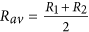, isHowever, when the atoms represent different isotopic pools with possibly different isotope ratios, the real clumped isotope ratio, Rcl, of doubly substituted isotopocules relative to the light isotopocules is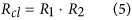The apparent statistical clumped isotope Δ is the relative difference between the real and the stochastically expected clumped isotope ratioThe clumped isotope composition is always negative, except for the case R1= R2, for which Δ = 0. Thus, when two atoms of the same element with different isotopic composition combine in a molecule, the resulting molecule will always have an apparent negative clumping signature. The black curve in Fig. 2a shows the size of this quadratic statistical negative isotope clumping according to Eq. (6). The negative clumping signal Δ does not depend on the absolute value of the underlying isotope ratios, but only on the relative difference of the isotope ratios. In the following we refer to this effect as “statistical clumped isotope signature”. Eq. 6 was first derived for the case of formation of molecular O2 in photosynthesis by Yeung et al.14, who indeed observed negative clumped isotope signals relative to the thermodynamically expected values for photosynthetic O2.

### Generalization: Molecules with three or more atoms of the same element – complete substitution

When a molecule contains three or more atoms of the same element, these atoms can generally represent populations with different isotope ratios R1, R2, … Rn. We first consider the case of full heavy-isotope substitution, which is the generalization of the two-atom case presented above. As it is not possible to independently measure the individual isotope ratios Ri of the indistinguishable atoms, the arithmetic average isotope ratio 18Rav(≈18Rbulk, see Supplementary Information) is assigned to each of the indistinguishable atoms for further calculationsAs the atoms are all assigned the same atomic isotope ratio Rav, the stochastically expected ratio of fully substituted isotopocules relative to non-substituted isotopocules from this population of atoms is the n-th power of Rav.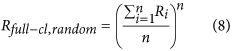This is the n-dimensional equivalent of replacing blue rectangle in Fig. 1 by the red square. The real (=observed) ratio of fully-substituted isotopocules is the product of all isotope ratios involved, which is identical to the n-th power of the geometric mean of the isotope ratios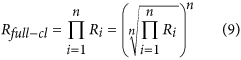Thus, the statistical clumped isotope signature for fully substituted isotopocules is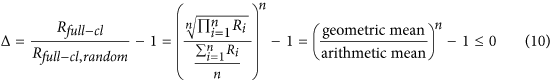This equation applies to any set of indistinguishable atoms in a molecule. Since the arithmetic mean is always larger or equal than the geometric mean, the statistical clumped isotope signal is always negative, except for the case where all ratios Ri are identical, in which case the arithmetic and geometric means are equal and thus Δ = 0. Figure 2 shows the variation of Δ with the relative difference of the isotope ratios in the 2-, 3-, 4- and 10-atom systems. For the cases illustrated in Fig. 2a, only one isotope ratio is varied and the isotope ratios of all other atoms are kept constant and identical. For the same relative difference in isotope ratio of a single atom, the clumping signal decreases with increasing number of atoms. Although the 10-atom case may not be of much practical use, it is included to emphasize the point that the statistical negative heavy isotope clumping does not require the heavy isotopes to be linked directly by a common chemical bond. For example, statistical negative D-D clumping in ethane (C2H6, Table 1) may involve pairs of hydrogen atoms at any of the 6 positions.

The isotope combinations presented in Fig. 2a all include the point where all isotope ratios are equal, which corresponds to Δ = 0‰. However, in general, the isotope ratios of the atoms at different positions are not equal. Figures 2b,c show the clumping signal for the 3- and 4-atom cases when one ratio is varied again and the other ratios are held constant, but at different values for the individual atoms. Now the situation that all isotope ratios are equal cannot occur and the parabola-shaped curves are shifted towards negative Δ values. The y-axis offset increases with increasing difference of the individual isotope ratios, thus with the heterogeneity of the isotopic composition of the individual indistinguishable atoms. The curves in Fig. 2b,c are selected 2-dimensional cross-sections of a multi-dimensional space, which illustrate the effect of varying one of the multiple isotope ratios relative to a fixed set of other ratios. In practice, a certain combination of isotope ratios among indistinguishable atoms will correspond to one single value of Δ and we will show below that the statistical clumped isotope signal Δ is a measure for the heterogeneity of the isotopic composition of indistinguishable atoms in a molecule.

### Molecules with three or more atoms of the same element – incomplete substitution

#### Clumping of two heavy isotopes in molecules with three indistinguishable atoms (e.g. 18O-18O or 17O-17O clumping in O3 or NO3)

As a first example we consider molecules with three indistinguishable atoms and calculate the clumping signature of double substituted isotopocules. The three atoms generally represent three isotopically different populations with isotope ratios R1, R2 and R3. However, as the atoms are indistinguishable, the individual ratios cannot be determined and for further calculations they are assigned the average isotope ratio, which can be determined from measurement of the single substituted isotopoculesThe stochastically expected isotope ratio of isotopocules with exactly two out of three possible heavy isotopes relative to the light isotopocules from a population of indistinguishable atoms with assigned isotope ratio Rav is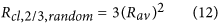The factor 3 gives the number of possible permutations of two heavy isotopes over three atom positions. The real probability for finding a molecule with exactly two heavy atoms from the three atoms with isotope ratios R1, R2 and R3 is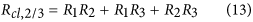Thus, the statistical clumped isotope signal for clumping of two out of 3 possible heavy isotopes, Δ2/3, is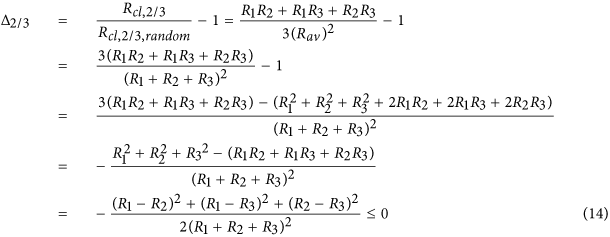Since all terms in the numerator and denominator are squares and thus positive, Δ is always negative except for the case R1 = R2 = R3 when Δ2/3 = 0. Some examples for the clumping of two out of three heavy isotopes in a molecule are shown in Fig. 3a. The solid line again includes the case where all ratios are identical and Δ = 0. The dashed and dotted lines show examples where one isotope ratio varies and the other ones are constant but different, so that the case that all are equal does not occur. Again, the dashed and dotted curves are shifted to more negative Δ values.

### Clumping of two heavy isotopes in molecules with four indistinguishable atoms (e.g. D-D clumping in CH4)

We now consider molecules with four indistinguishable atoms and calculate the clumping signature of doubly-substituted isotopocules. The four atoms generally represent isotopically distinct pools with isotope ratios R1, R2, R3 and R4. As the ratios cannot be determined individually, they are assigned the average atomic isotope ratio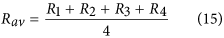The stochastically expected probability for forming a molecule with exactly two out of four possible heavy isotopes from a population of indistinguishable atoms with assigned isotope ratio Rav is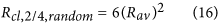The factor 6 again gives the number of possible permutations of 2 heavy isotopes over 4 atom positions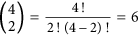. However, the real probability to form an isotopocule with exactly two heavy atoms from the four atoms with different isotope ratios R1, R2, R3 and R4 isThus, the clumped isotope signal Δ isSince Δ can again be expressed as a negative sum of squares, it is always negative, except for R1 = R2 = R3 = R4 where Δ2/4 = 0. Some examples for the statistical clumped isotope effect of two out of four heavy isotopes in a molecule are shown in Fig. 3b. An important example for this case is the D-D clumping in methane. The reservoirs that supply the different hydrogen atoms in the formation of methane can vary considerably and significant apparent statistical anti-clumping is expected.

### Generalization: Clumping of m heavy isotopes in molecules with n indistinguishable atoms

For the general case of n indistinguishable atoms that represent isotopic pools with isotope ratios R1, R2, … Rn, we assign again the arithmetic mean isotope ratio Rav to each of the atoms.The stochastically expected ratio of isotopocules with exactly m out of a possible n heavy atoms relative to the light isotopocules from a population of indistinguishable atoms with this average heavy isotope ratio Rav is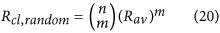The real ratio of isotopocules with m heavy isotopes relative to non-substituted isotopocules (Rcl) from n atoms with isotope ratios R1, R2, … Rn is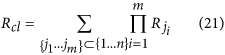Thus, the clumped isotope signal Δ isEq. 22 is the most general equation to calculate statistical negative isotope clumping in any multi-isotope system. In the case where all ratios Ri are identical, Rji = Rav, the numerator becomes identical to the denominator because the number of possible subsets {j1, … jm} out of {1, … n} is equal to the binomial coefficientand the products reduce to the factors (Rav)m. In this case, Δ = 0.

### More than 2 stable isotopes: 17O-18O clumping for oxygen

So far we have formally only treated molecules with two stable isotopes. Oxygen has three stable isotopes 16O, 17O and 18O. Clumping of heavy isotopes of the same sort (i.e., clumping of multiple 17O atoms or multiple 18O atoms in one molecule) follows the examples and general rules outlined above (see Table 1). However, it is also possible that the heavy 17O and 18O atoms clump together in one molecule.

### O2 and other molecules with two indistinguishable O atoms

In the case of molecular O2, the apparent statistical clumping signal for 17O-18O clumping was derived in Yeung et al.14. Since the isotope ratios of the individual O atoms cannot be determined individually, they are assigned the average heavy isotope ratios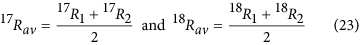The stochastically expected (random) ratio of molecules with one 17O and one 18O isotope relative to 16O16O from this population of O atoms isThe real probability for forming 17O18O molecules from two atoms with isotope ratio 17R1, 18R1 and 17R2, 18R2 is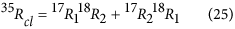Therefore,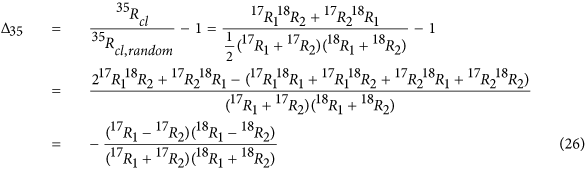For normal mass dependent fractionation, where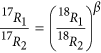ref. 24, with three-isotope exponent β ≈ 0.53 ref. 25, 17R1 and 18R1 are either both smaller or both larger than 17R2 and 18R2, thus again the clumped isotope signature 35Δ is always <0 ( = 0 if iR1 and iR2 are identical). This can also be shown by inserting the mass dependent fractionation relation as follows: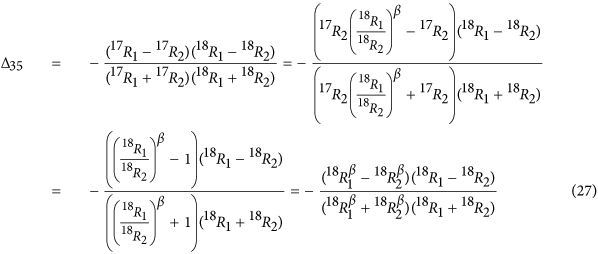As the atoms do not need to share the same bond to generate statistical isotope clumping, the equation also applies to 17O-18O clumping of other molecules with 2 O atoms (importantly 17O-18O clumping in CO2). The resulting negative clumping signature is shown in Fig. 4.

### Heavy isotope clumping for three indistinguishable oxygen atoms (e.g. O3)

When a molecule has three indistinguishable (or not distinguished) oxygen atoms, we assign the average ratios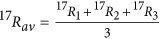and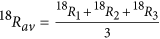to each of the atoms. The statistical clumping signatures for complete 17O or 18O substituted isotopocules and for 17O-17O and 18O-18O clumping can be derived according to the formalism derived above. Here we also consider the remaining combinations where 17O and 18O clump together in one molecule.

Since there are 3! = 6 different possibilities to distribute the three distinguishable atoms (16O, 17O and 18O) over the three positions, the stochastically expected clumping isThe real clumping is calculated by considering explicitly all 6 configurations of 16O, 17O and 18O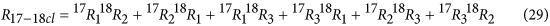and thus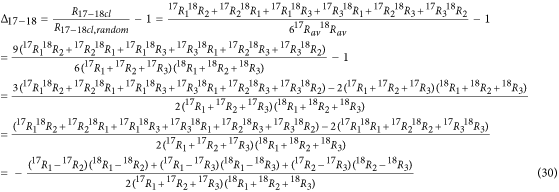As argued above for O2, for normal mass dependent fractionation, 17Ri and 18Ri are either both smaller or both larger than 17Rj and 18Rj, thus Δ is always < 0 (=0 if all ratios are identical). Inserting the mass dependent fractionation relationagain, Eq. 30 can be transformed toThe corresponding values of Δ are shown in Fig. 5 together with all other possible isotope clumping combinations for 17O and 18O isotopes in O3. These are always calculated as the relative difference of the explicitly calculated clumped isotope ratio iRcl from the stochastically expected heavy isotope ratio iRcl,random (Equation 30). For example, for 17O-17O-18O clumping there are three possible configurations to distribute the atoms (17O, 17O and 18O) over the three positions, so the stochastically expected clumping isThe real clumping is calculated by considering explicitly all 3 configurations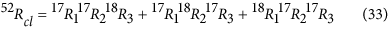and thus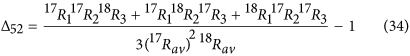The corresponding equation for 17O-18O-18O clumping is easily derived by exchanging 17O and 18O.

Important examples of molecules with three oxygen atoms are O3 and the nitrate ion NO3. For O3, the central and terminal O atoms can be distinguished with suitable techniques2,26,27,28,29. In these cases, statistical anti-clumping only occurs when the indistinguishable terminal O atoms are involved (Table 1). In many cases, however, the isotopic composition of O3 is determined without position information30,31,32,33 and in this case the atoms can be treated as effectively indistinguishable and need to be treated according to the formalism developed here.

### Statistical clumped isotope signals and the heterogeneity of the isotope ratios of indistinguishable atoms

The derivations above show that statistical combination of indistinguishable atoms in a molecule leads to apparent negative clumped isotope signals. The size of the apparent negative clumping in the molecule increases with increasing difference in isotopic composition between the individual atoms, so it may actually contain scientifically relevant information. In order to investigate this further, we created random (within a certain range) sets of isotope ratios for all multi-isotope systems with up to 5 indistinguishable atoms and calculated the apparent negative multi-isotope clumping signature Δ. Figure 6 shows that for each multi-isotope set all these random sets of isotope ratios yield Δ values that fall on distinct curves when Δ is plotted versus the relative standard deviation of the individual isotope ratios. For each of the multi-isotope clumping combinations with m out of n heavy isotopes, the curves can be parameterized as:Note that the Δ values in Fig. 6 are plotted as ‰, so the factor 1/2 in Eq. 35 corresponds to 500‰ in the fit curves.

In the case of m = 2 (2 heavy isotopes in any multi-isotope system) the fit is perfect, but for more heavy atoms clumping in one molecule there is some scatter around these fit lines. This originates from the fact that there is no fixed analytical relation between the arithmetic and geometric means. Figure 7 shows the relative deviation of the explicitly calculated Δ values and the approximation using Eq. 35 for the randomly chosen sets of isotope ratios with known standard deviation and average values. The outer envelopes of the point clouds for each multi-isotope system define the error with which the statistical clumped isotope signal Δ in a molecule can be predicted from Eq. 35 when the standard deviation and the mean of the individual isotope ratios are known.

Scientifically, the opposite relationis more attractive, since the isotopic variability among indistinguishable atoms in a molecule is usually not known, but Δ may be measurable14. This means that measurement of the statistical clumped isotope anomaly Δ could provide a novel tracer to determine the heterogeneity (quantified by the standard deviation) of the isotopic pools of indistinguishable atoms in a molecule. For example, in the 2-isotope system O2, a Δ value of 1.5‰ below the thermodynamically expected value as measured by Yeung et al.14 would indicate a relative standard deviation in the isotope ratios of the two O atoms of about 5.5% (Fig. 6, blue curve).

Figure 8 shows the relative error that is made when Eq. 36 is used to calculate the relative standard deviation of the isotope ratios from the Δ value for randomly chosen sets of (known) isotope ratios. The outer envelope for each isotope system quantifies the error with whichfor a group of indistinguishable atoms can be derived from Δ. Above Δ values of −1.5‰ the relative error inis generally below 1% and above Δ values of −5‰ the relative error is still only 2%. Thus, whereas we are not able to measure the isotopic composition of individual indistinguishable atoms, the apparent statistical isotope clumping provides a means to obtain information about the heterogeneity of the isotope ratios with quite good precision.